# Class 3 IMO previous years Level 2 question papers - Set 8

Check these Class 3 IMO Level 2 questions from previous years Maths Olympiad exams.

For our paid members, we offer comprehensive benefits, including access to detailed explanations for all questions in our Chapterwise Test Series and Mock Test Series. Our paid members have the opportunity to attempt more than a dozen tests for each chapter covered in the SOF IMO syllabus for Class 3. They can attempt each test multiple times to enhance their learning experience.Our courses can be bought at our BUY COURSES section.

Our Mock Tests for Class 3 IMO follow the exact pattern of SOF IMO in terms of questions, marking and time alloted for completion. This helps the students to experience of the real time exam environment and assess their exam preparedness. Since we follow the same marking system , the scores obtained at the end of each test will help identify students' weaknesses and assist them in improving.

Olympiadtester has displayed a few Chapterwise tests and Mock tests in the FREE SECTION.

1) Find the perimeter of the shaded figure.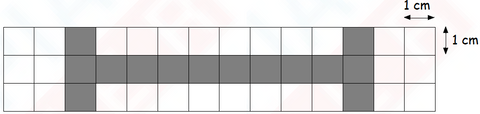OPTIONS

1. 24 cm
2. 28 cm
3. 29 cm
4. 30 cm

2) Which figure has the largest area?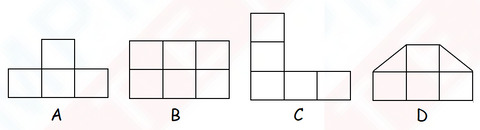OPTIONS

1. A
2. B
3. C
4. D

3) Which one of the following figures consists of 3 right angles only?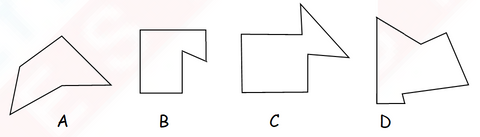OPTIONS

1. A
2. B
3. C
4. D

4) Arrange the given angles in order of size, beginning with the greatest.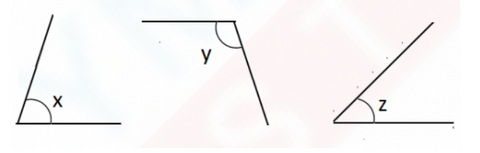OPTIONS

1. Angle x, Angle y, Angle z
2. Angle y, Angle z, Angle x
3. Angle x, Angle z, Angle y
4. Angle y, Angle x, Angle z

5) The area of each square is 4m2. What is the total area of the figure below?OPTIONS

1. 52 m2
2. 48 m2
3. 44 m2
4. 12 m2

6) Which of the following 2 figures have the same number of right angles in them?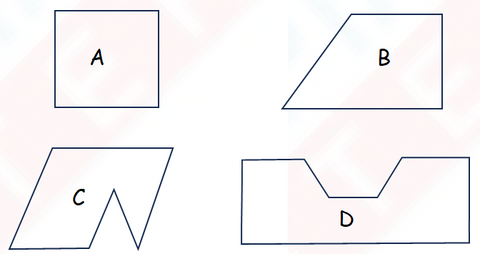OPTIONS

1. A and B
2. B and C
3. A and D
4. C and D

7 How many angles are there in the object below?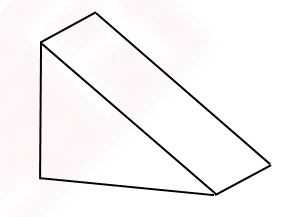OPTIONS

1. 7
2. 10
3. 14
4. 18

8) Study figures A and B carefully. Which figure has a greater area?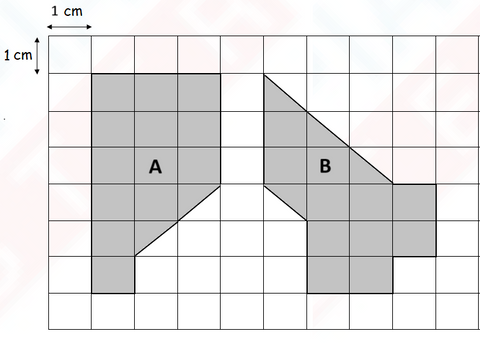OPTIONS

1. Figure A
2. Figure B
3. Both figures have the same area
4. Cannot be determined without further information

9) The figure shown below is made up of five similar rectangles 12 cm by 6 cm.
Find the perimeter of the figure.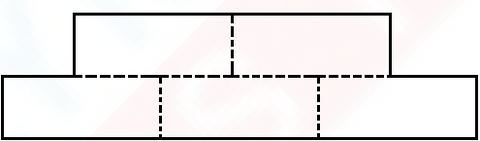OPTIONS

1. 99 cm
2. 89 cm
3. 96 cm
4. 98 cm

10) Look at the figure below. Which pair of lines is parallel?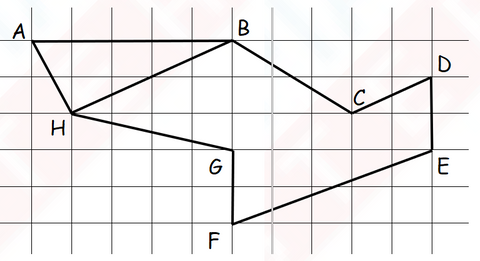OPTIONS

1. HB // CD
2. HG // BC
3. CD // FE
4. AH // BC

10) The figure below is made up Square X and Rectangle Y. Find the perimeter of the figure.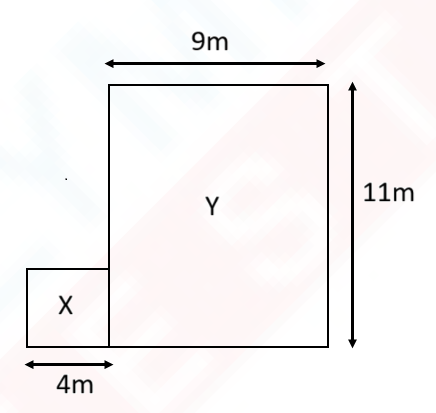11) The figure below is made up of 6 similar squares of sides 3 cm. What is the perimeter of the figure?OPTIONS

1. 33 cm
2. 29 cm
3. 37 cm
4. 42 cm

12) How many angles inside the figure shown below are greater than a right angle?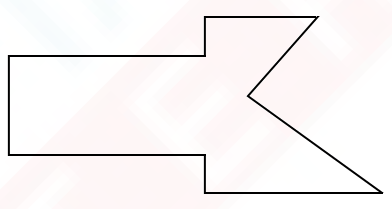Ans: _______________

13) Find the perimeter of the figure below.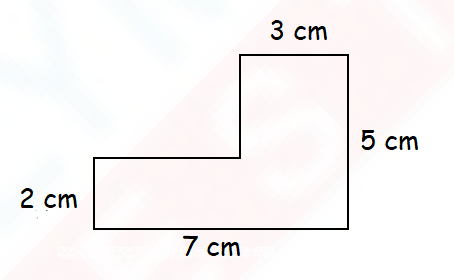Ans: _______________ cm

14) Find the area of the shaded figure.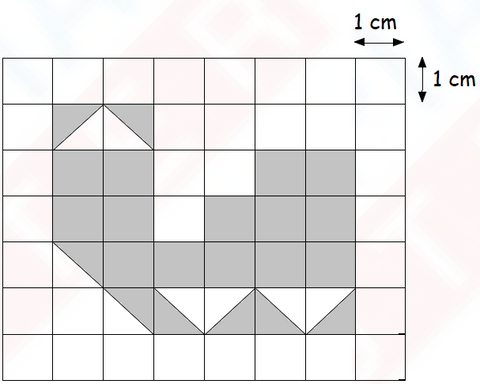Ans: ________________ cm2

15)Anuj draw this figure on a piece of paper.

a) How many angles are there inside the figure?
b) How many angles inside the figure are greater than a right angle?Olympiadtester has displayed a few Chapterwise tests and Mock tests in the FREE SECTION.